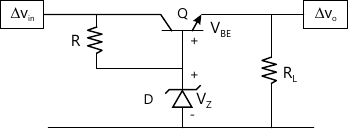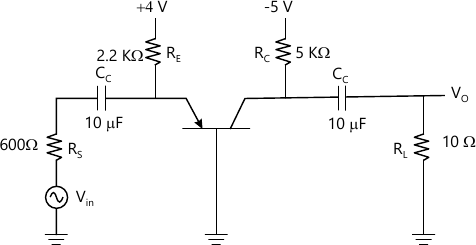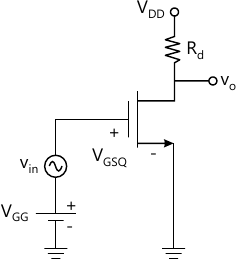MORE IN Analog Electronics - 1
MU Electronics and Telecom Engineering (Semester 3)
Analog Electronics - 1
December 2012
Total marks: --
Total time: --
INSTRUCTIONS
(1) Assume appropriate data and state your reasons
(2) Marks are given to the right of every question
(3) Draw neat diagrams wherever necessary

1 (a) Design for Supply voltage Vcc, Collector resistor RC, Emitter resistor RE. voltage divider biasing resistor R1 & R2 of a single stage RC coupled CE audio amplifier for peak output voltage 3V at 5k? load, Av ?100 and SICO ?10.
10 M
1 (b) Answer the following question related to design of single stage CE audio frequency voltage amplifier
1. Upper limit and lower limit on choice of power supply voltage VCC
2. Upper limit on the choice of collector resistor RC
3. Lower limit on selection of emitter resistor RE
4. Experimentally how will you determine input impedance and output impedance of CE without measuring input and output current.
10 M

2 (a) Design single stage RC coupled common source (CS) audio amplifier employing JFET type BFW11 typical to provide a voltage gain of Av?10 at peak output voltage of Vo = 4.5V. load resistor of 120k?, supply voltage of 20V with biasing circuit to provide IDQ =3 mA .
10 M
2 (b) For the above designed circuit with source resistor "RS' unbypassed. Determine voltage gain. Input impedance. output impedance and output voltage for input voltage of 20Vpp.
10 M

3 (a) Design a full wave rectifier dc supply using centre tapped transformer with two diodes to give dc output voltage at 150 volts to a variable resistive load. The load current expected is 50 ± 10 mA with ripple factor not to exceed 0.07. Use L-C fi
10 M
3 (b) Explain operation of the circuit shown in figure A with detailed funcion of resistor 'R' zener diode 'D', and BJT 'Q' in above circuit10 M

4 (a) (i) The best location for setting a Q-point on d.c. load line of an FET amplifier is at
a) Saturation point
b) Cut-off point
c) Mid-point
d) None of these
2 M
4 (a) (ii) When transistors are used in digital circuits they usually operate in the:
a) Active region
b) Break down region
c) Saturation region
d) Saturation & cut-off region
2 M
4 (a) (iii) When an input delta of 2 V produces a transconductance or 1.5 ms, what is the drain current delta?
a) 666 mA ?
b) 3mA
c) 0.75 mA
d) 0.5 mA
2 M
4 (a) (iv) Introducing a resistor in the emitter of a common emitter amplifier stabilizes the dc operating point against variations in: -
a) Only the temperature
b) Only' the ? of the transistor
c) Both temperature and ?
d) None of the above
2 M
4 (a) (v) A Voltage regulator has a no-load output of 18 V and a full- load output of 17.3 V. The percent load regulations is
a) 0.25%
b) 96.1%
c) 4.05%
d) 1.04%
2 M
Explain the following (Any two):
4 (b) (i) Graphical determination of FET parameters
5 M
4 (b) (ii) Features of IGBT
5 M
4 (b) (iii) BJT as a Switch:
5 M
4 (b) (iv) Power MOSFET
5 M

5 (a) Discuss using the concept of a load line-superimposed On the transistor characteristics. how a simple common emitter circuit can amplify a time varying signal
10 M
5 (b) The following parameters are obtained from certain JFET data sheet: VGsoff =- 8 V and IDSS=5 mA. Determine the values of lD, for each value of VGS ranging from 0 V to - 8 Vin 1V steps: Plot the transfer characteristic curve for the same data.
10 M

6 (a) For the network shown in Figure below, determine the following with IVBEonl == 0.7V and ?=1OO 1. ICQ and IEQ 2. Input &.Output impedance 3. Voltage gain 4. Current gain 5. List disadvantages of circuit shown10 M
6 (b) Determine the small signal voltage gain of a MOSFET circuit with VGSQ=2.12V, VDD -5V, Rd =2.5k?. VTN=1V. Kn=0.8 mA/V2 and ?=0.02, V-1 (body effect coefficient). Assume transistor is biased in the saturation region.10 M

Explain in brief and sketch experimental setup to determine ?
7 (a) Output characteristic of CE BJT voltage amplifier
4 M
7 (b) Line regulation of BJT shunt regulator
4 M
7 (c) Forward characteristics of SCR
4 M
7 (d) Transfer characteristics of JFET
4 M
7 (e) UJT- Characteristics
4 M

More question papers from Analog Electronics - 1